# NCERT Solution (Ex - 3.1) - Chapter 3: Understanding Quadrilaterals, Maths, Class 8 Notes | Study Additional Documents & Tests for Class 8 - Class 8

## Class 8: NCERT Solution (Ex - 3.1) - Chapter 3: Understanding Quadrilaterals, Maths, Class 8 Notes | Study Additional Documents & Tests for Class 8 - Class 8

The document NCERT Solution (Ex - 3.1) - Chapter 3: Understanding Quadrilaterals, Maths, Class 8 Notes | Study Additional Documents & Tests for Class 8 - Class 8 is a part of the Class 8 Course Additional Documents & Tests for Class 8.
All you need of Class 8 at this link: Class 8

Exercise 3.1

Question 1:
Given here are some figures: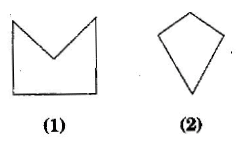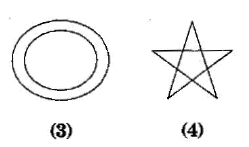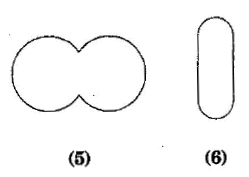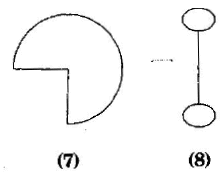Classify each of them on the basis of the following:

(a) Simple curve
(b) Simple closed curve
(c) Polygon
(d) Convex polygon
(e) Concave polygon

(a) Simple curve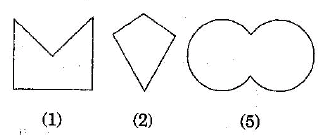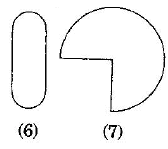(b) Simple closed curve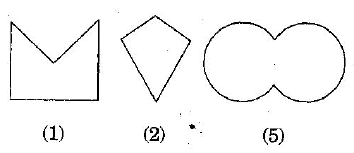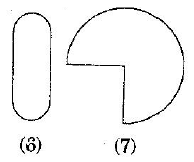(c) Polygons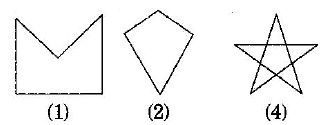(d) Convex polygons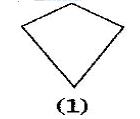(e) Concave polygon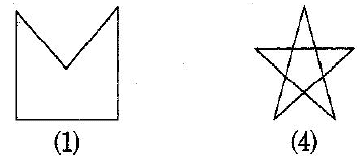Question 2:
How many diagonals does each of the following have?
(b) A regular hexagon
(c) A triangle

(a) A convex quadrilateral has two diagonals.
Here, AC and BD are two diagonals.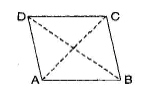(b) A regular hexagon has 9 diagonals.
Here, diagonals are AD, AE, BD, BE, FC, FB, AC, EC and FD.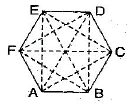(c) A triangle has no diagonal.

Question 3:
What is the sum of the measures of the angles of a convex quadrilateral? Will this property hold if the quadrilateral is not convex? (Make a non-convex quadrilateral and try)

Let ABCD is a convex quadrilateral, then we draw a diagonal AC which divides the quadrilateral in two triangles.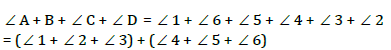= 180+180o                              [By Angle sum property of triangle]
= 360o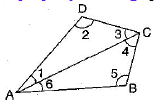Hence, the sum of measures of the triangles of a convex quadrilateral is 360o.
Yes, if quadrilateral is not convex then, this property will also be applied.

Let ABCD is a non-convex quadrilateral and join BD, which also divides the quadrilateral in two triangles.
Using angle sum property of triangle,
In ΔABD,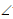1 +2 +3 = 180o ……….(i)
In ΔBDC,4 +5 +6 = 180o ……….(i)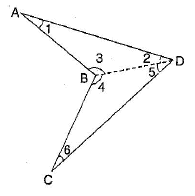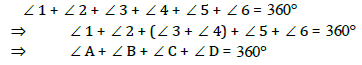Hence proved.

Question 4:
Examine the table. (Each figure is divided into triangles and the sum of the angles deduced from that.)

 Figure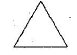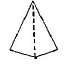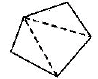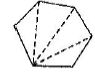Side 3 4 5 6 Angle sum 1 x 1800 = (3- 2) x 1800 2x1800 = (4-2)xl800 3x1800 = (5-2)xl800 4x1800 = (6-2)xl800

What can you say about the angle sum of a convex polygon  with number of sides?

(a) When n = 7, then
Angle sum of a polygon = (n - 2)x 180o = (7 - 2)x180o = 5x180o = 900o
(b) When n = 8, then
Angle sum of a polygon = (n - 2)x 180o = (8 - 2)x180o = 6x180o =1080o
(c) When n = 10, then
Angle sum of a polygon = (n - 2)x 180o = (10 - 2)x180o = 8x180o =1440o
(d) When n = n, then
Angle sum of a polygon = (n - 2)x 180o

Question 5:
What is a regular polygon? State the name of a regular polygon of:
(a) 3 sides
(b) 4 sides
(c) 6 sides

A regular polygon: A polygon having all sides of equal length and the interior angles of equal size is known as regular polygon.
(i) 3 sides - Polygon having three sides is called a triangle.
(ii) 4 sides - Polygon having four sides is called a quadrilateral.
(iii) 6 sides - Polygon having six sides is called a hexagon.

Question 6:
Find the angle measures x in the following figures: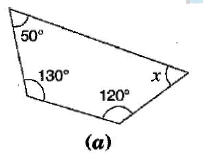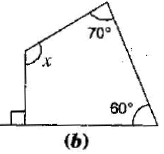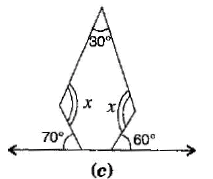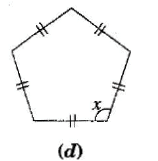(a) Using angle sum property of a quadrilateral,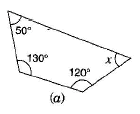50o+130o+120o+ x = 360o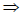300o+ x = 360ox = 360- 300ox = 60o

(b) Using angle sum property of a quadrilateral,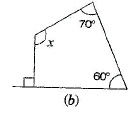90o+60o+70o+ x = 360o220o+ x = 360ox = 360o-220ox =140o

(c) First base interior angle = 180o- 70o =110o
Second base interior angle = 180o- 60o = 120o
There are 5 sides, n = 5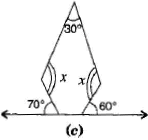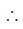Angle sum of a polygon = (n - 2)x180o
= (5-2)x 180= 3x180= 540o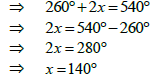(d) Angle sum of a polygon = (n - 2)x180o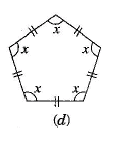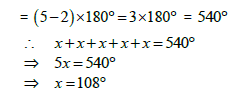Hence each interior angle is 108o

Question 7:
(a) Find x + y + z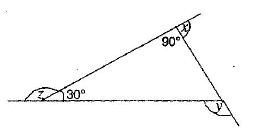(b) Find x+y+z+w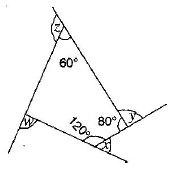(a) Since sum of linear pair angles is 180o.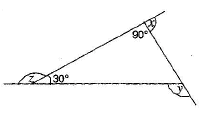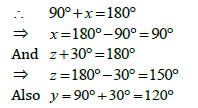[Exterior angle property]x+y+x=90o+120o+150o=360o

(b) Using angle sum property of a quadrilateral,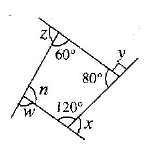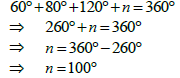Since sum of linear pair angles is 180.w+100 +180o ……….(i)
x+120o +180o ……….(ii)
y +80o +180o ……….(iii)
z +60o +180o ……….(iv)
Adding eq. (i), (ii), (iii) and (iv),x + y + z + w+ 100o +120o + 80o + 60o +180o +180o +180o +180ox + y + z + w+ 360o = 720ox + y + z + w = 720o - 360ox + y + z + w = 360o

The document NCERT Solution (Ex - 3.1) - Chapter 3: Understanding Quadrilaterals, Maths, Class 8 Notes | Study Additional Documents & Tests for Class 8 - Class 8 is a part of the Class 8 Course Additional Documents & Tests for Class 8.
All you need of Class 8 at this link: Class 8Use Code STAYHOME200 and get INR 200 additional OFF

## Additional Documents & Tests for Class 8

43 docs

Track your progress, build streaks, highlight & save important lessons and more!

,

,

,

,

,

,

,

,

,

,

,

,

,

,

,

,

,

,

,

,

,

,

,

,

,

,

,

;